Better, Faster, Stronger Sequence Tagging Constituent Parsers

# 介绍

• 太长的短语预测错误率高。
• 输出空间太大导致label稀疏性。
• 贪心解码导致的错误传播。

• 采用融合了相对编码和绝对编码的动态编码。
• 将预测任务分解为多个子任务。
• 采用辅助任务和策略梯度。

# 三大问题以及解决方法

## 过长短语预测的高错误率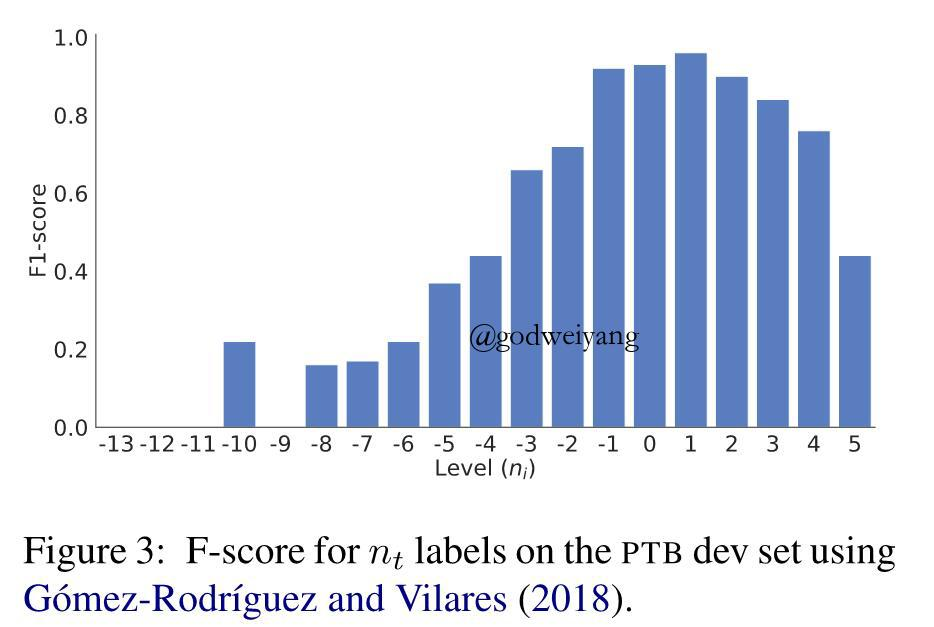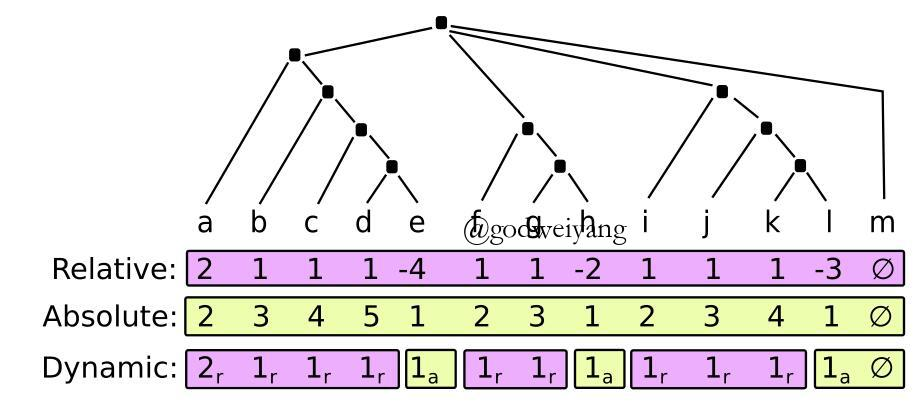• 绝对值$n_t’ \leq 3$，也就是说CA的个数不能超过3个，这样也是为了降低数据的稀疏性。
• 相对值$n_t \leq -2$，也就是说将上图中准确率比较低的那些负数值全部用绝对值替代了，在句法树中表现为$w_{t+1}$所在的子树比$w_t$低两层以上。

## 输出空间太大导致label稀疏性

$\mathcal{L} = \mathcal{L}_n + \mathcal{L}_c + \mathcal{L}_u$

## 贪心解码导致的错误传播$\mathcal{L} = \mathcal{L}_n + \mathcal{L}_c + \mathcal{L}_u + \beta \sum_a \mathcal{L}_a$

$p(tree) = \prod\limits_{t = 1}^T {\pi ({l_t}|{s_t};\theta )}$

$\mathcal R = \sum\limits_{tree} { {\mathcal R_{tree}}p(tree)}$

$\begin{array}{l}\Delta \mathcal R = \sum\limits_{tree} { {\mathcal R_{tree}}\Delta p(tree)} \\ = \sum\limits_{tree} {p(tree){\mathcal R_{tree}}\frac{ {\Delta p(tree)}}{ {p(tree)}}} \\ = \sum\limits_{tree} {p(tree){\mathcal R_{tree}}\Delta \log p(tree)} \\ = {\mathbb{E}_{tree \sim p}}({\mathcal R_{tree}}\Delta \log p(tree))\end{array}$

$\begin{array}{l}\Delta \mathcal R = {\mathbb{E}_{tree \sim p}}({\mathcal R_{tree}}\Delta \log p(tree))\\ = {\mathbb{E}_{tree \sim p}}({\mathcal R_{tree}}\Delta \log \prod\limits_{t = 1}^T {\pi ({l_t}|{s_t};\theta )} )\\ = {\mathbb{E}_{tree \sim p}}(\sum\limits_{t = 1}^T { {\mathcal R_{tree}}\Delta \log \pi ({l_t}|{s_t};\theta )} )\\ \approx \frac{1}{N}\sum\limits_{i = 1}^N {\sum\limits_{t = 1}^T {\mathcal R_{i}\Delta \log \pi ({l_t}|{s_t};\theta )} } \end{array}$

$\Delta \mathcal R \approx \frac{1}{N}\sum\limits_{i = 1}^N {\sum\limits_{t = 1}^T {\Delta \log \pi ({l_t}|{s_t};\theta )({\mathcal R_i} - {B_i})} }$

$\Delta \mathcal R \approx \frac{1}{N}\sum\limits_{i = 1}^N {\sum\limits_{t = 1}^T {\Delta \log \pi ({l_t}|{s_t};\theta )({\mathcal R_i} - {B_i}) + \beta \Delta H(\pi ({l_t}|{s_t};\theta ))} }$

$\Delta \mathcal R \approx \frac{1}{N}\sum\limits_{i = 1}^N {\sum\limits_{t = 1}^T {\Delta (\log \pi ({l_t}|{s_t};\theta ) + N)({\mathcal R_i} - {B_i}) + \beta \Delta H(\pi ({l_t}|{s_t};\theta ) + N)} }$

# 实验结果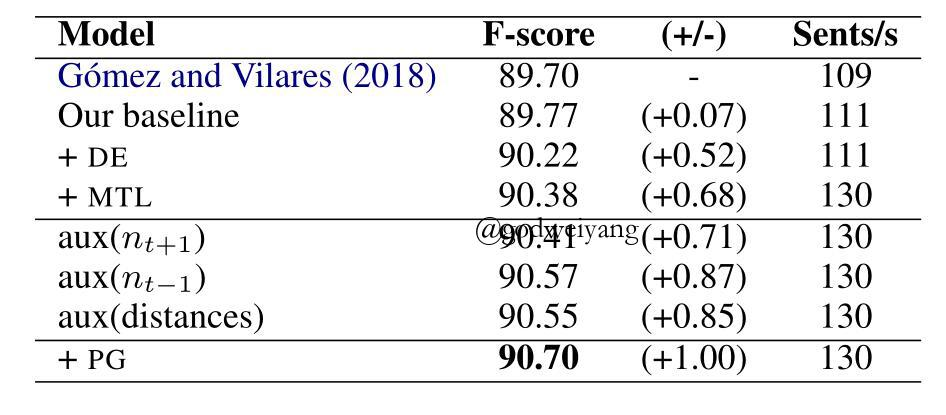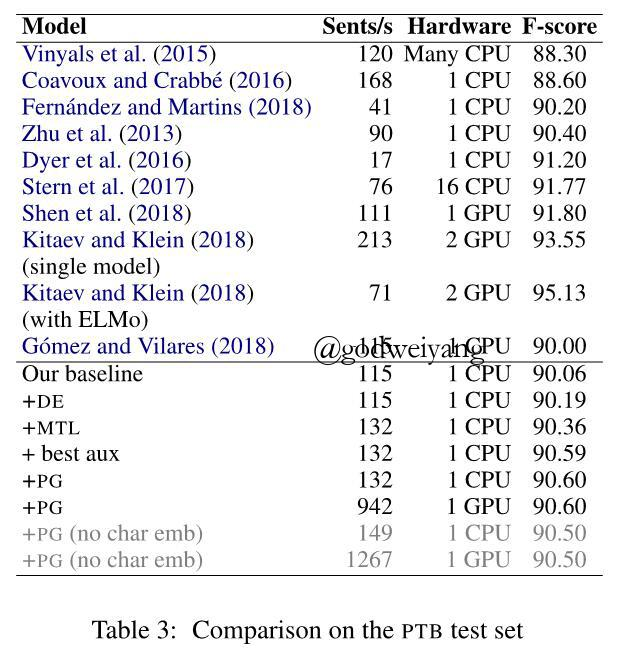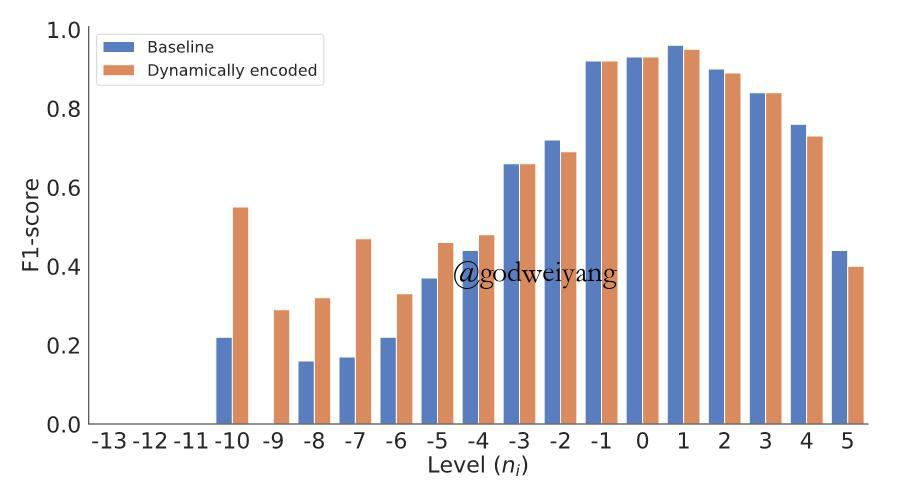# 总结

转载规则

《Better, Faster, Stronger Sequence Tagging Constituent Parsers》 韦阳 采用 知识共享署名 4.0 国际许可协议 进行许可。
上一篇Neural Language Modeling by Jointly Learning Syntax and Lexicon

2019-03-31Constituent Parsing as Sequence Labeling

2019-03-11
目录